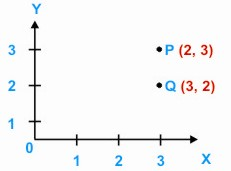HOME MATH DICTIONARY DOWNLOAD FEEDBACK DISCLAIMER
 Question: What is Ordered Pair ? Answer: When a pair of numbers such as (2,3) is represented as a point on graph paper, then the order of the numbers is important.In this case the first number 2, is the x-coordinate and the second number, 3, is the y-coordinate. See point P. The pair (3, 2) is represented by Q whose x-coordinate is 3 and y-coordinate. When the order of two numbers is taken into account they form an ordered pair. In contrast, consider a game where someone wants to score a total of 5 from the roll of two dice. It makes no difference whether a 2 follows a 3 or a 3 follows a 2.